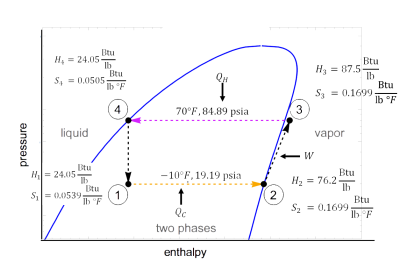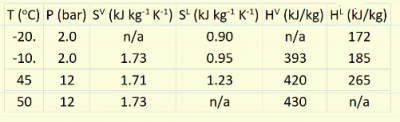LearnChemE

#### Refrigeration Cycle: Example Problem

Try to solve these problems before watching the solutions in the screencasts.

##### Example Problem 1

A refrigeration cycle using freon-12 is shown in the log pressure versus enthalpy plot. This cycle uses a throttle valve. The enthalpy and entropy values in the plot are from tables for freon-12. Calculate:
1. Heat transferred at low temperature and at high temperature.
2. Work input to refrigerator.
3. The coefficient of performance (COP) for the cycle.
4. The COP if the compressor is only 70% efficient.##### Example Problem 2

Consider a vapor-compression refrigeration cycle that uses R134a as the working fluid. The condenser, which yields saturated liquid, is operated at 45C; the evaporator, which yields saturated vapor, is operated at -10.C. The compressor is 80.% efficient. Using data from the table below, determine:
1. The amount of cooling per kg of R134a
2. The amount of heat rejected per kg of R134a
3. The coefficient of performance.